• No products in the cart.

# 203.3.10 Pruning a Decision Tree in R

## Pruning

In previous section, we studied about The Problem of Over fitting the Decision Tree

• Growing the tree beyond a certain level of complexity leads to overfitting
• In our data, age doesn’t have any impact on the target variable.
• Growing the tree beyond Gender is not going to add any value. Need to cut it at Gender
• This process of trimming trees is called Pruning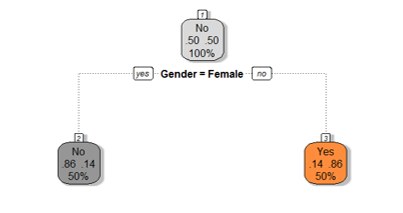``````buyers_model1<-rpart(Bought ~ Gender, method="class", data=Train,control=rpart.control(minsplit=2))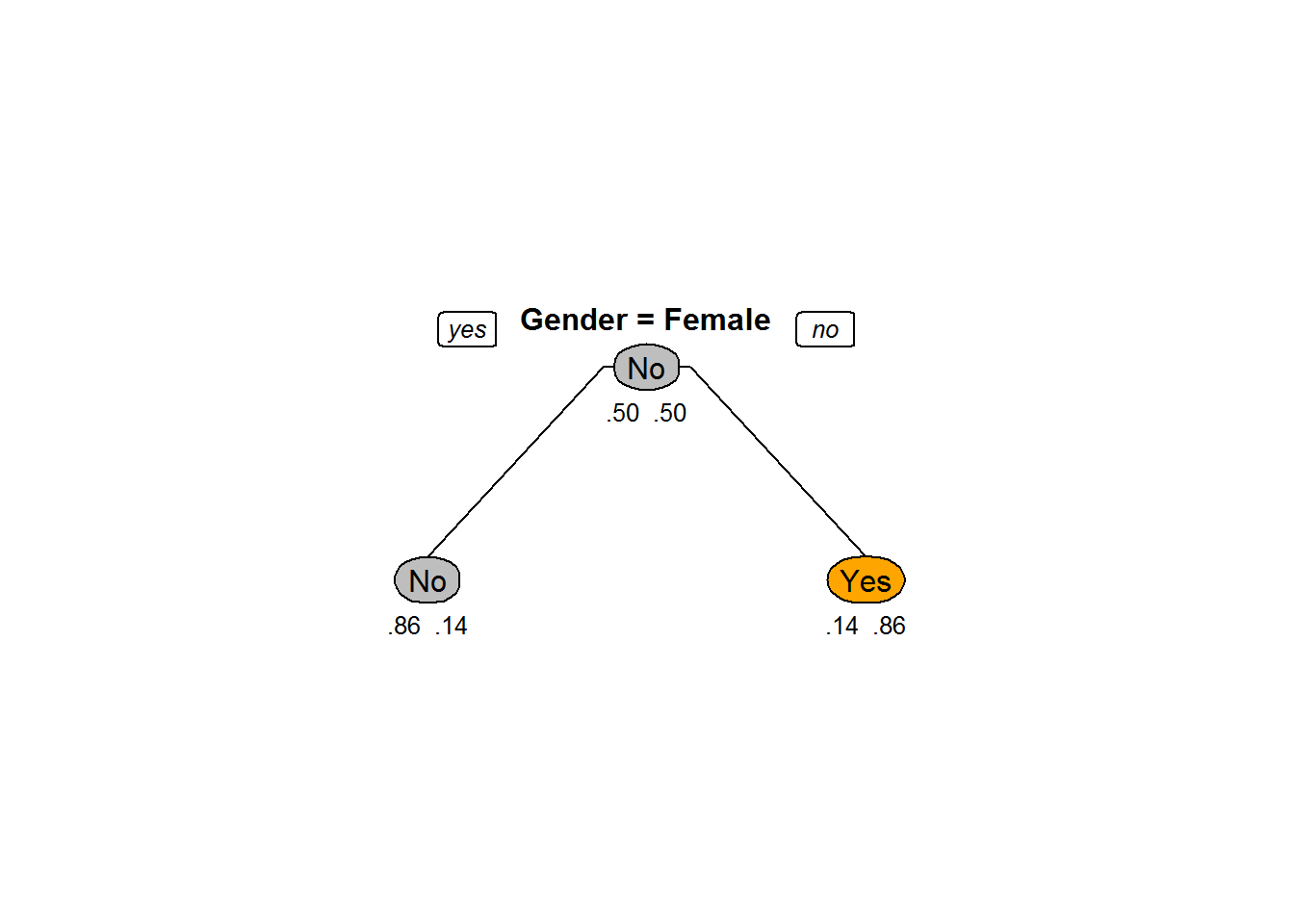### Pruning to Avoid Overfitting

• Pruning helps us to avoid overfitting
• Generally it is preferred to have a simple model, it avoids overfitting issue
• Any additional split that does not add significant value is not worth while.
• We can use Cp – Complexity parameter in R to control the tree growth

## Complexity Parameter

• Complexity parameter is used to mention the minimum improvement before proceeding further.
• It is the amount by which splitting a node improved the relative error.
• For example, in a decision tree, before splitting the node, the error is 0.5 and after splitting the error is 0.1 then the split is useful, where as if the error before splitting is 0.5 and after splitting it is 0.48 then split didn’t really help
• User tells the program that any split which does not improve the fit by cp will likely be pruned off
• This can be used as a good stopping criterion.
• The main role of this parameter is to avoid overfitting and also to save computing time by pruning off splits that are obviously not worthwhile
• It is similar to Adj R-square. If a variable doesn’t have a significant impact then there is no point in adding it. If we add such variable adj R square decreases.
• The default is of cp is 0.01.

### Code-Tree Pruning and Complexity Parameter

``````Sample_tree<-rpart(Bought~Gender+Age, method="class", data=Train, control=rpart.control(minsplit=2, cp=0.001))
Sample_tree``````
``````## n= 14
##
## node), split, n, loss, yval, (yprob)
##       * denotes terminal node
##
##  1) root 14 7 No (0.5000000 0.5000000)
##    2) Gender=Female 7 1 No (0.8571429 0.1428571)
##      4) Age>=20 4 0 No (1.0000000 0.0000000) *
##      5) Age< 20 3 1 No (0.6666667 0.3333333)
##       10) Age< 11.5 2 0 No (1.0000000 0.0000000) *
##       11) Age>=11.5 1 0 Yes (0.0000000 1.0000000) *
##    3) Gender=Male 7 1 Yes (0.1428571 0.8571429)
##      6) Age>=47 3 1 Yes (0.3333333 0.6666667)
##       12) Age< 52 1 0 No (1.0000000 0.0000000) *
##       13) Age>=52 2 0 Yes (0.0000000 1.0000000) *
##      7) Age< 47 4 0 Yes (0.0000000 1.0000000) *``````
``````Sample_tree_1<-rpart(Bought~Gender+Age, method="class", data=Train, control=rpart.control(minsplit=2, cp=0.1))
Sample_tree_1``````
``````## n= 14
##
## node), split, n, loss, yval, (yprob)
##       * denotes terminal node
##
## 1) root 14 7 No (0.5000000 0.5000000)
##   2) Gender=Female 7 1 No (0.8571429 0.1428571) *
##   3) Gender=Male 7 1 Yes (0.1428571 0.8571429) *``````
• The default is 0.01.

### Choosing Cp and Cross Validation Error

• We can choose Cp by analyzing the cross validation error.
• For every split we expect the validation error to reduce, but if the model suffers from overfitting the cross validation error increases or shows negligible improvement
• We can either rebuild the tree with updated cp or prune the already built tree by mentioning the old tree and new cp value
• printcp(tree) shows the
• Training error , cross validation error and standard deviation at each node.

### Code – Choosing Cp

• Cp display the results
``printcp(Sample_tree)``
``````##
## Classification tree:
## rpart(formula = Bought ~ Gender + Age, data = Train, method = "class",
##     control = rpart.control(minsplit = 2, cp = 0.001))
##
## Variables actually used in tree construction:
##  Age    Gender
##
## Root node error: 7/14 = 0.5
##
## n= 14
##
##         CP nsplit rel error  xerror    xstd
## 1 0.714286      0   1.00000 1.71429 0.18704
## 2 0.071429      1   0.28571 0.28571 0.18704
## 3 0.001000      5   0.00000 0.71429 0.25612``````

### Code – Cross Validation Error

• cross-validation results
``plotcp(Sample_tree)``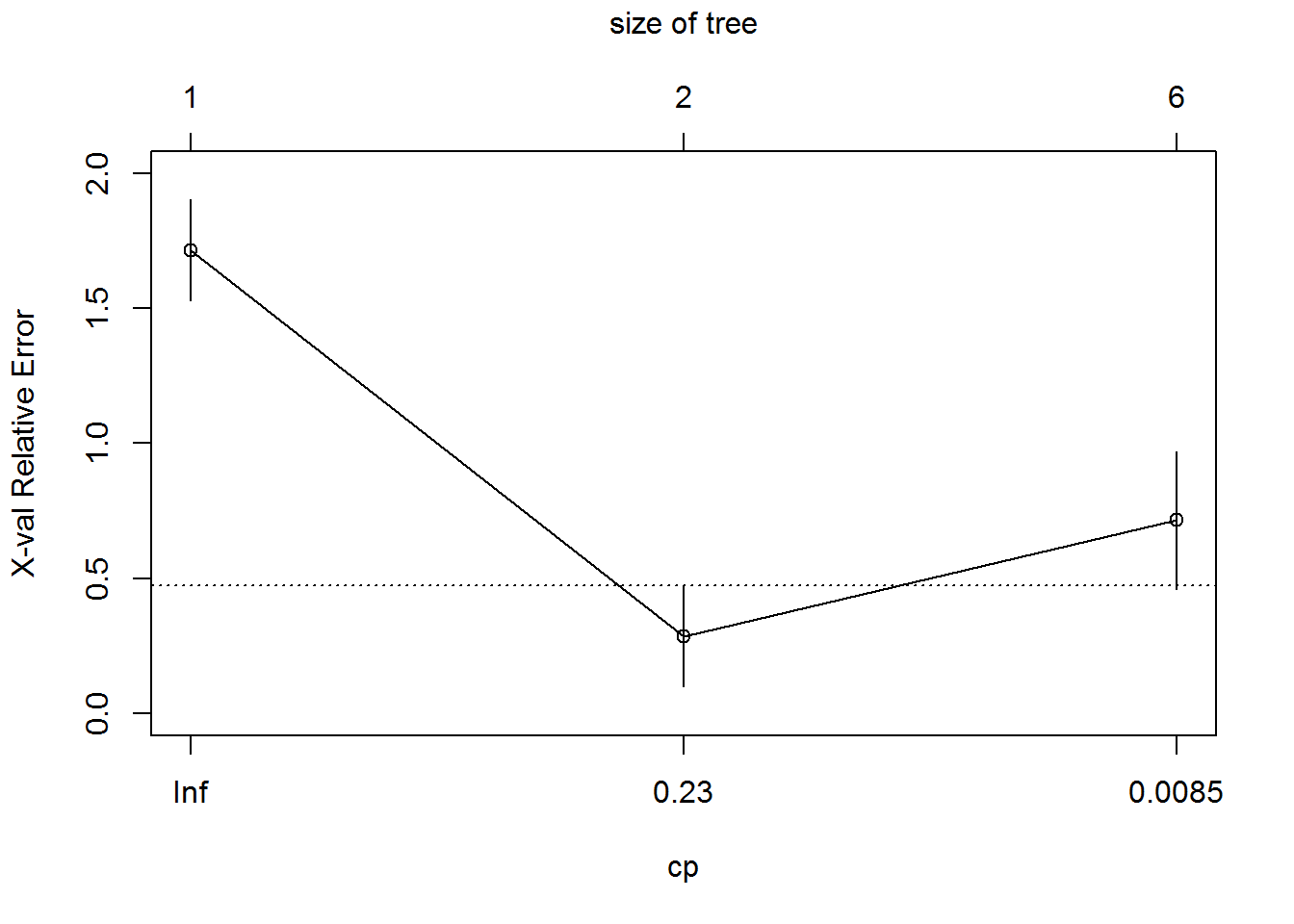### New Model with Selected Cp

``````Sample_tree_2<-rpart(Bought~Gender+Age, method="class", data=Train, control=rpart.control(minsplit=2, cp=0.23))
Sample_tree_2``````
``````## n= 14
##
## node), split, n, loss, yval, (yprob)
##       * denotes terminal node
##
## 1) root 14 7 No (0.5000000 0.5000000)
##   2) Gender=Female 7 1 No (0.8571429 0.1428571) *
##   3) Gender=Male 7 1 Yes (0.1428571 0.8571429) *``````

### Plotting the Tree

``prp(Sample_tree_2,box.col=c("Grey", "Orange")[Sample_tree_2\$frame\$yval],varlen=0,faclen=0, type=1,extra=4,under=TRUE)``### Post Pruning the Old Tree

``````Pruned_tree<-prune(Sample_tree,cp=0.23)
prp(Pruned_tree,box.col=c("Grey", "Orange")[Sample_tree\$frame\$yval],varlen=0,faclen=0, type=1,extra=4,under=TRUE)``````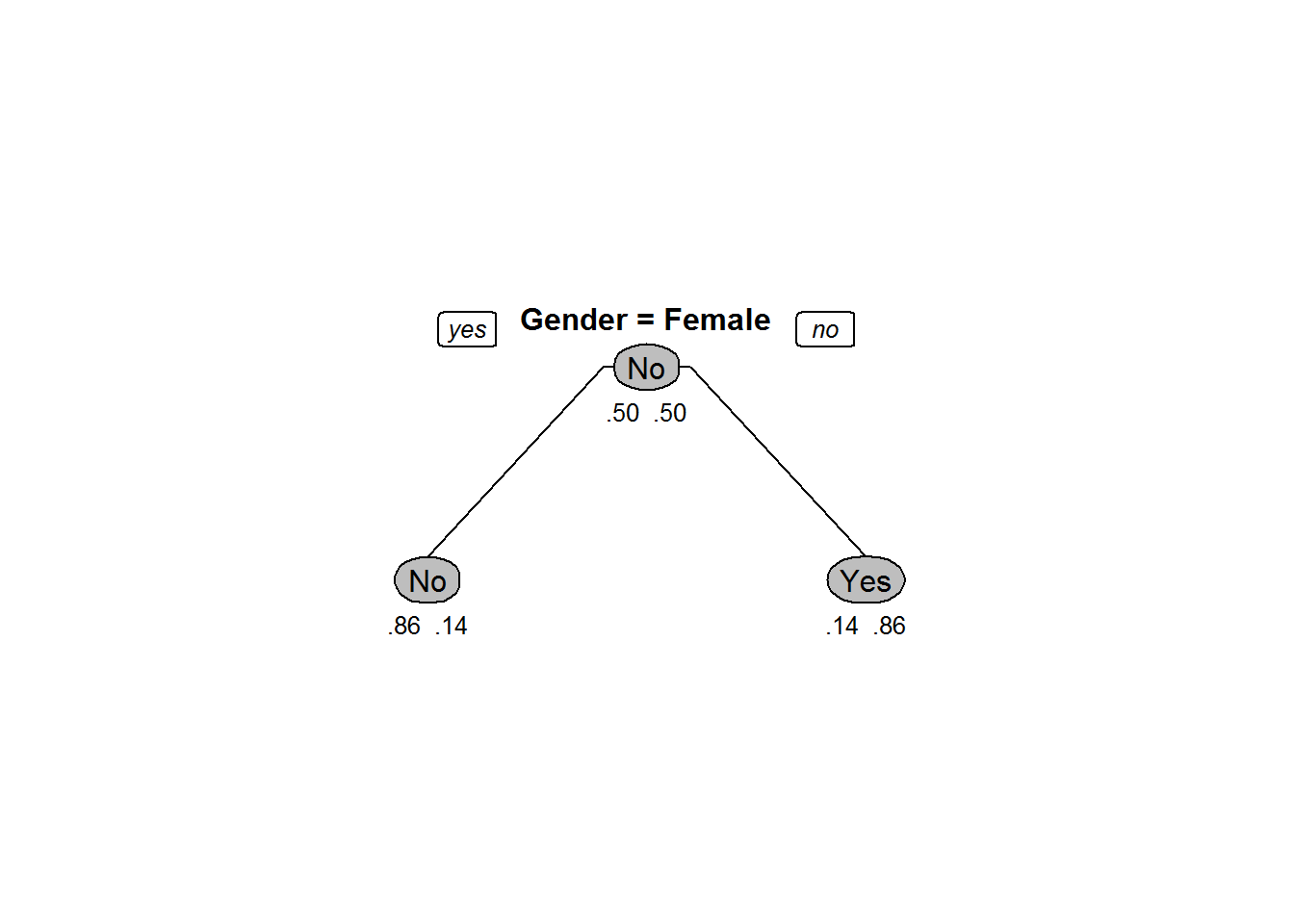### Code-Choosing Cp

``````Ecom_Tree<-rpart(Overall_Satisfaction~Region+ Age+ Order.Quantity+Customer_Type+Improvement.Area, method="class", control=rpart.control(minsplit=30,cp=0.001),data=Ecom_Cust_Survey)
printcp(Ecom_Tree)``````
``````##
## Classification tree:
## rpart(formula = Overall_Satisfaction ~ Region + Age + Order.Quantity +
##     Customer_Type + Improvement.Area, data = Ecom_Cust_Survey,
##     method = "class", control = rpart.control(minsplit = 30,
##         cp = 0.001))
##
## Variables actually used in tree construction:
##  Age              Customer_Type    Improvement.Area Order.Quantity
##  Region
##
## Root node error: 5401/11812 = 0.45725
##
## n= 11812
##
##          CP nsplit rel error  xerror      xstd
## 1 0.8035549      0   1.00000 1.00000 0.0100245
## 2 0.0686910      1   0.19645 0.19645 0.0057537
## 3 0.0029624      2   0.12775 0.12775 0.0047193
## 4 0.0022218      5   0.11887 0.12572 0.0046839
## 5 0.0018515      7   0.11442 0.12127 0.0046053
## 6 0.0014812      8   0.11257 0.11757 0.0045385
## 7 0.0010000      9   0.11109 0.11776 0.0045419``````

Code-Choosing Cp

``plotcp(Ecom_Tree)``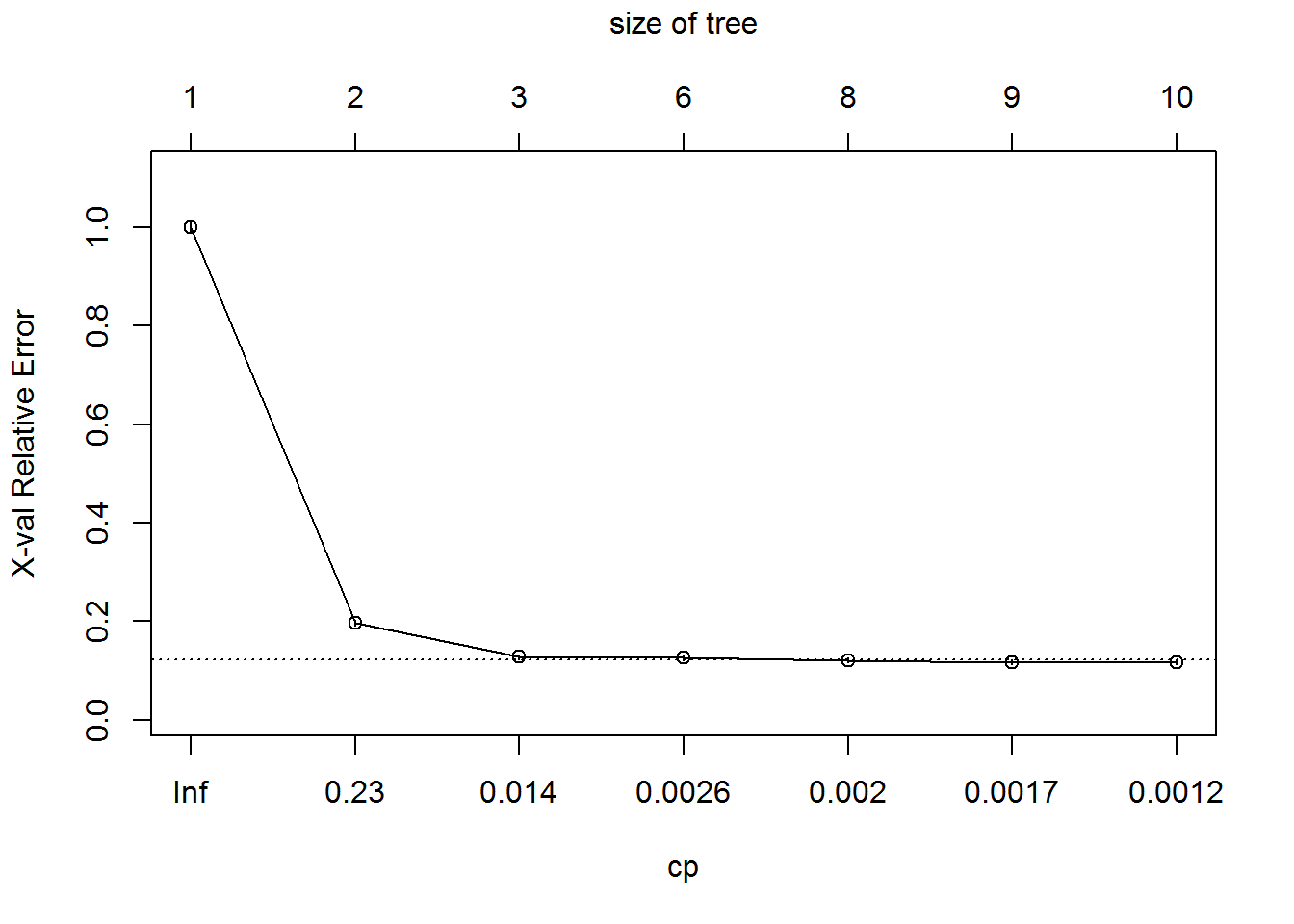– Choose Cp as 0.0029646

### Code – Pruning

``````Ecom_Tree_prune<-prune(Ecom_Tree,cp=0.0029646)
Ecom_Tree_prune``````
``````## n= 11812
##
## node), split, n, loss, yval, (yprob)
##       * denotes terminal node
##
## 1) root 11812 5401 Dis Satisfied (0.542753132 0.457246868)
##   2) Order.Quantity< 40.5 7404 1027 Dis Satisfied (0.861291194 0.138708806)
##     4) Age>=29.5 7025  652 Dis Satisfied (0.907188612 0.092811388) *
##     5) Age< 29.5 379    4 Satisfied (0.010554090 0.989445910) *
##   3) Order.Quantity>=40.5 4408   34 Satisfied (0.007713249 0.992286751) *``````

### Plot – Beofre and After Pruning

``prp(Ecom_Tree,box.col=c("Grey", "Orange")[Ecom_Tree\$frame\$yval],varlen=0,faclen=0, type=1,extra=4,under=TRUE)``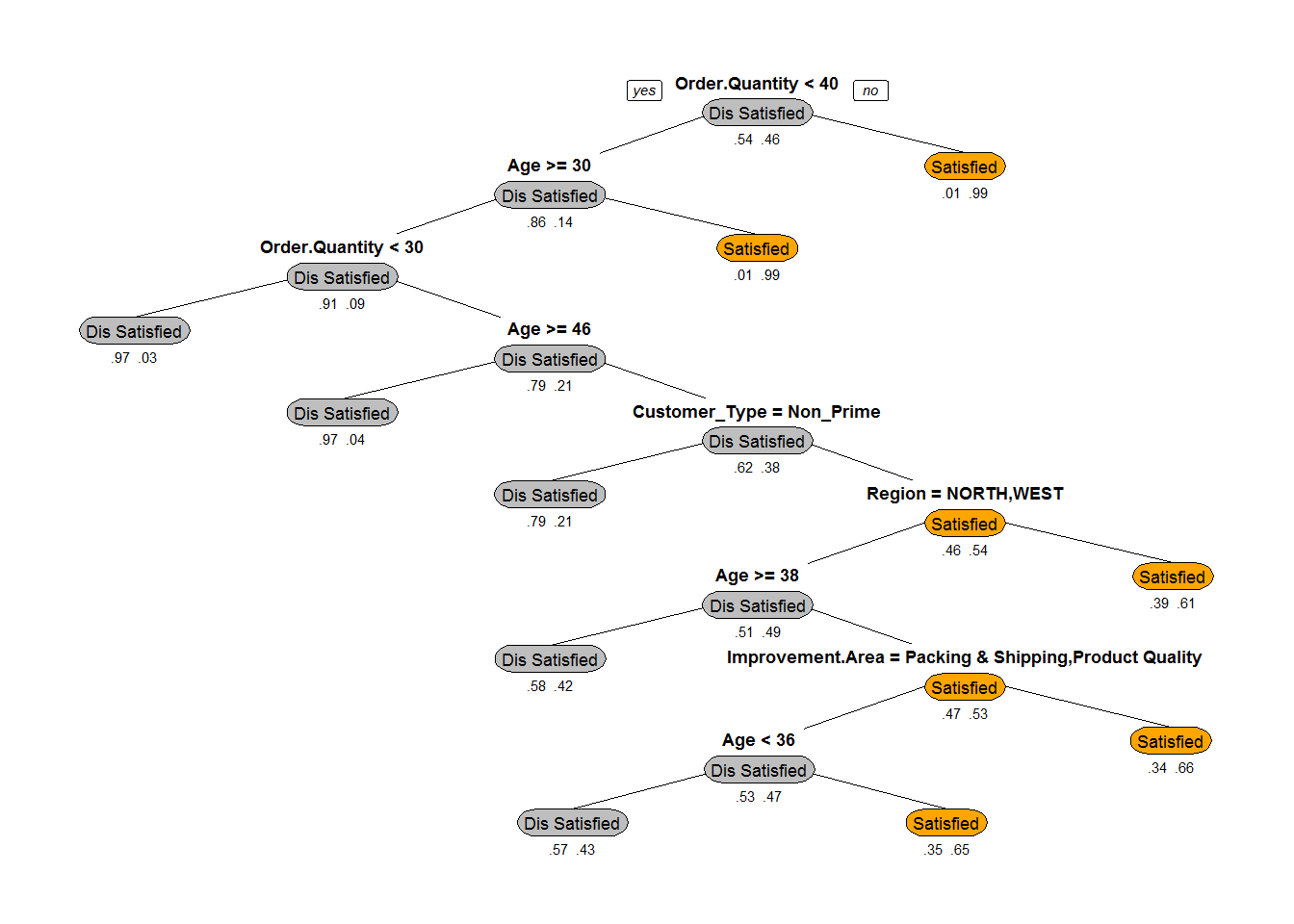***

``prp(Ecom_Tree_prune,box.col=c("Grey", "Orange")[Ecom_Tree\$frame\$yval],varlen=0,faclen=0, type=1,extra=4,under=TRUE)``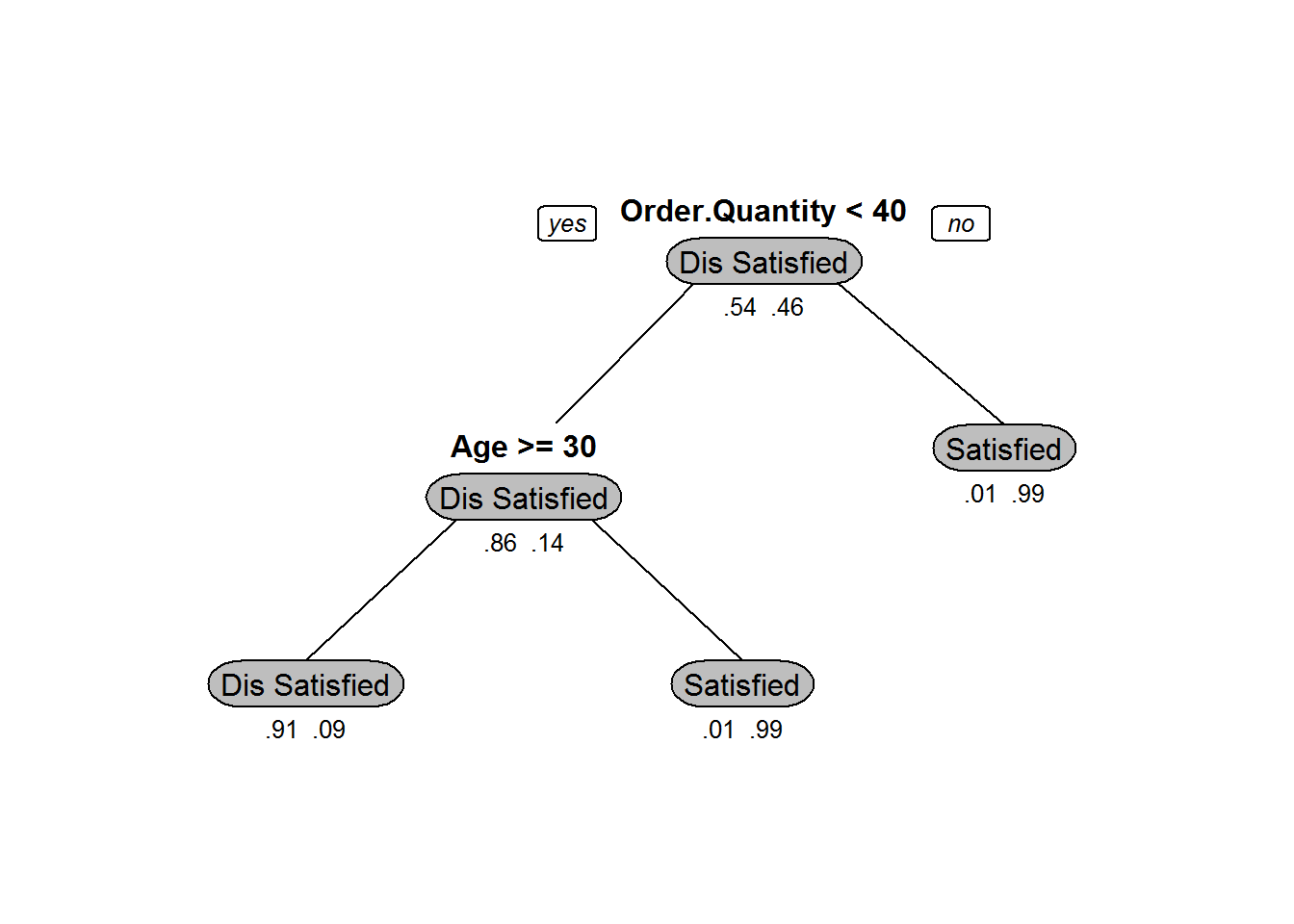### Two Types of Pruning

• Pre-Pruning:
• Building the tree by mentioning Cp value upfront
• Post-pruning:
• Grow decision tree to its entirety, trim the nodes of the decision tree in a bottom-up fashion

The next post is about Tree Building and Model Selection.

20th June 2017
0 responses on "203.3.10 Pruning a Decision Tree in R"